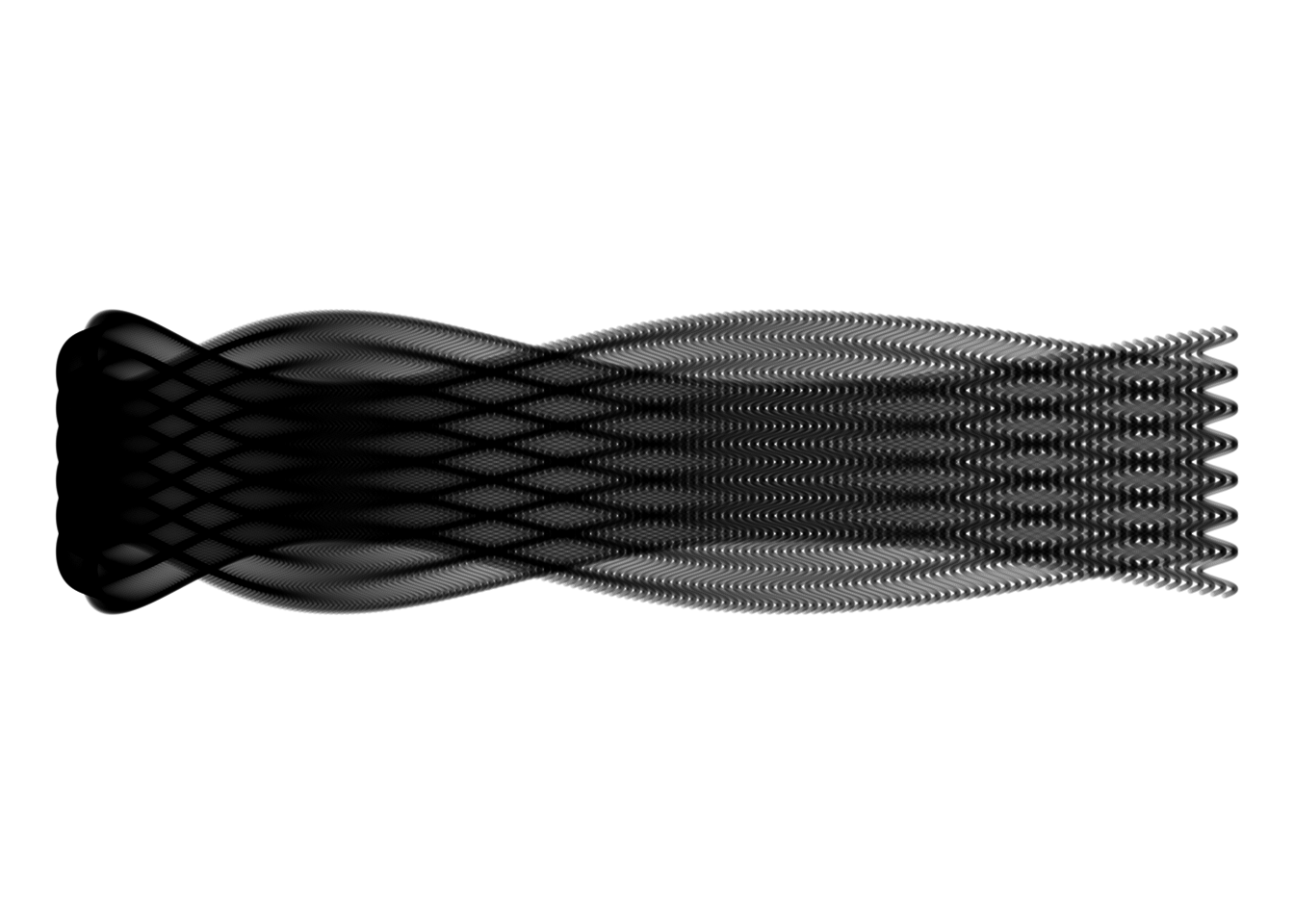# Inspiration

Basic Abstract Spiral

``````  ## Data
ab.seq <- as.data.frame(seq(from = 1,
to = 100,
by = 1))

ab.seq\$V1 <- rep(0, times = 100)

colnames(ab.seq) <- c("ab", "V1")

## Plot
ggplot() +
geom_polygon(data = (ab.seq + 250), mapping = aes(x = 1:100, y = V1),
alpha = 0.5) +

geom_point(data = (ab.seq - 150), mapping = aes(x = 1:100, y = ab)) +
geom_point(data = (ab.seq - 100), mapping = aes(x = 1:100, y = ab)) +
geom_point(data = (ab.seq - 50), mapping = aes(x = 1:100, y = ab)) +
geom_point(data = (ab.seq), mapping = aes(x = 1:100, y = ab)) +
geom_point(data = (ab.seq + 50), mapping = aes(x = 1:100, y = ab)) +
geom_point(data = (ab.seq + 100), mapping = aes(x = 1:100, y = ab)) +
geom_point(data = (ab.seq + 150), mapping = aes(x = 1:100, y = ab)) +
ylim(-100, 250) +
theme_void() +
coord_polar()``````Colourful Spiral V1

``````## Data
ab.seq.2 <- as.data.frame(seq(from = 0,
to = 99.99,
by = 0.01))

ab.seq.2\$ab2 <- seq(from = 99.99,
to = 0,
by = -0.01)

ab.seq.2\$col <- rep(seq(from = 1,
to = 5,
by = 1))

ab.seq.2\$V1 <- rep(0, time = 10000)

colnames(ab.seq.2) <- c("ab", "ab2", "col", "V1")

ggplot() +
geom_polygon(data = (ab.seq.2 + 200),
mapping = aes(x = 1:10000, y = (V1)), fill = "grey") +

geom_point(data = (ab.seq.2 - 100),
mapping = aes(x = 1:10000, y = ab, colour = ab)) +
geom_point(data = (ab.seq.2 - 50),
mapping = aes(x = 1:10000, y = ab, colour = ab)) +
geom_point(data = (ab.seq.2),
mapping = aes(x = 1:10000, y = ab, colour = ab)) +
geom_point(data = (ab.seq.2 + 50),
mapping = aes(x = 1:10000, y = ab, colour = ab)) +
geom_point(data = (ab.seq.2 + 100),
mapping = aes(x = 1:10000, y = ab, colour = ab)) +
geom_point(data = (ab.seq.2 + 150),
mapping = aes(x = 1:10000, y = ab, colour = ab)) +
geom_point(data = (ab.seq.2 + 200),
mapping = aes(x = 1:10000, y = ab, colour = ab)) +

geom_point(data = (ab.seq.2 - 75),
mapping = aes(x = 1:10000, y = ab, colour = ab)) +
geom_point(data = (ab.seq.2 - 25),
mapping = aes(x = 1:10000, y = ab, colour = ab)) +
geom_point(data = (ab.seq.2),
mapping = aes(x = 1:10000, y = ab, colour = ab)) +
geom_point(data = (ab.seq.2 + 25),
mapping = aes(x = 1:10000, y = ab, colour = ab)) +
geom_point(data = (ab.seq.2 + 75),
mapping = aes(x = 1:10000, y = ab, colour = ab)) +
geom_point(data = (ab.seq.2 + 125),
mapping = aes(x = 1:10000, y = ab, colour = ab)) +
geom_point(data = (ab.seq.2 + 175),
mapping = aes(x = 1:10000, y = ab, colour = ab)) +

ylim(0, 200) + xlim(0, 10000) +
theme_void() +
theme(legend.position = "none") +
coord_polar()``````Colourful Spiral V2

``````## Data
ab.seq.2 <- as.data.frame(seq(from = 0,
to = 99.99,
by = 0.01))

ab.seq.2\$ab2 <- seq(from = 99.99,
to = 0,
by = -0.01)

ab.seq.2\$col <- (rep(seq(from = 1,
to = 5,
by = 1)))

ab.seq.2\$V1 <- rep(0, time = 10000)

colnames(ab.seq.2) <- c("ab", "ab2", "col", "V1")

ggplot() +
geom_polygon(data = (ab.seq.2 + 200),
mapping = aes(x = 1:10000, y = (V1)), fill = "lightblue") +

geom_point(data = (ab.seq.2 - 100),
mapping = aes(x = 1:10000, y = ab, colour = ab)) +
geom_point(data = (ab.seq.2 - 50),
mapping = aes(x = 1:10000, y = ab, colour = ab)) +
geom_point(data = (ab.seq.2),
mapping = aes(x = 1:10000, y = ab, colour = ab)) +
geom_point(data = (ab.seq.2 + 50),
mapping = aes(x = 1:10000, y = ab, colour = ab)) +
geom_point(data = (ab.seq.2 + 100),
mapping = aes(x = 1:10000, y = ab, colour = ab)) +
geom_point(data = (ab.seq.2 + 150),
mapping = aes(x = 1:10000, y = ab, colour = ab)) +
geom_point(data = (ab.seq.2 + 200),
mapping = aes(x = 1:10000, y = ab, colour = ab)) +

geom_point(data = (ab.seq.2 - 75),
mapping = aes(x = 1:10000, y = ab, colour = ab)) +
geom_point(data = (ab.seq.2 - 25),
mapping = aes(x = 1:10000, y = ab, colour = ab)) +
geom_point(data = (ab.seq.2),
mapping = aes(x = 1:10000, y = ab, colour = ab)) +
geom_point(data = (ab.seq.2 + 25),
mapping = aes(x = 1:10000, y = ab, colour = ab)) +
geom_point(data = (ab.seq.2 + 75),
mapping = aes(x = 1:10000, y = ab, colour = ab)) +
geom_point(data = (ab.seq.2 + 125),
mapping = aes(x = 1:10000, y = ab, colour = ab)) +
geom_point(data = (ab.seq.2 + 175),
mapping = aes(x = 1:10000, y = ab, colour = ab)) +

low = "purple", high = "lightblue") +

ylim(0, 200) + xlim(0, 10000) +
theme_void() +
theme(legend.position = "none") +
coord_polar()``````Sin Celtic Circlular Weave

``````fs.seq <- as.data.frame(seq(from = 1,
to = 51.3,
by = 0.25))

fs.seq\$alpha <- rep(0, times = 202)

colnames(fs.seq) <- c("fs", "alpha")

ggplot() +
geom_polygon(data = fs.seq,
mapping = aes(x = 1:202, y = (alpha - 2))) +
geom_polygon(data = fs.seq,
mapping = aes(x = 1:202, y = (alpha + 2)), alpha = 0.2) +
geom_polygon(data = fs.seq,
mapping = aes(x = 1:202, y = (alpha + 4)), alpha = 0.2) +

geom_point(data = fs.seq,
mapping = aes(x = 1:202, y = (sin(fs) - 3))) +
geom_point(data = fs.seq,
mapping = aes(x = 1:202, y = (-sin(fs) - 3))) +

geom_point(data = fs.seq,
mapping = aes(x = 1:202, y = sin(fs))) +
geom_point(data = fs.seq,
mapping = aes(x = 1:202, y = -sin(fs))) +

geom_point(data = fs.seq,
mapping = aes(x = 1:202, y = (sin(fs) + 3))) +
geom_point(data = fs.seq,
mapping = aes(x = 1:202, y = (-sin(fs) + 3))) +

ylim(-10, 10) +
theme_void() +
coord_polar() ``````Colourful cos Celtic Circlular Weave

``````fs.seq <- as.data.frame(seq(from = 1,
to = 51.3,
by = 0.25))

fs.seq\$alpha <- rep(0, times = 202)

colnames(fs.seq) <- c("fs", "alpha")

ggplot() +
geom_polygon(data = fs.seq,
mapping = aes(x = 1:202, y = (alpha - 2)),
alpha = 0.2, fill = "purple") +
geom_polygon(data = fs.seq,
mapping = aes(x = 1:202, y = (alpha + 2)),
alpha = 0.2, fill = "purple") +
geom_polygon(data = fs.seq,
mapping = aes(x = 1:202, y = (alpha + 4)),
alpha = 0.2, fill = "purple") +

geom_point(data = fs.seq,
mapping = aes(x = 1:202, y = ((cos(fs)-7))),
alpha = 0.8, colour = "blue") +
geom_point(data = fs.seq,
mapping = aes(x = 1:202, y = ((-cos(fs)-7))),
alpha = 0.8, colour = "red") +

geom_point(data = fs.seq,
mapping = aes(x = 1:202, y = (cos(fs) - 3)),
alpha = 0.8, colour = "blue") +
geom_point(data = fs.seq,
mapping = aes(x = 1:202, y = (-cos(fs) - 3)),
alpha = 0.8, colour = "red") +

geom_point(data = fs.seq,
mapping = aes(x = 1:202, y = cos(fs)),
alpha = 0.5, colour = "blue") +
geom_point(data = fs.seq,
mapping = aes(x = 1:202, y = -cos(fs)),
alpha = 0.5, colour = "red") +

geom_point(data = fs.seq,
mapping = aes(x = 1:202, y = (cos(fs) + 3)),
alpha = 0.2, colour = "blue") +
geom_point(data = fs.seq,
mapping = aes(x = 1:202, y = (-cos(fs) + 3)),
alpha = 0.2, colour = "red") +

ylim(-10, 10) +
theme_void() +
coord_polar()``````Square Weaves

``````kw.seq <- (seq(from = -10,
to = 10,
by = 0.05)) %>%
expand.grid(x = ., y = .)

## Celtic Square Weave

ggplot() +
geom_point(data = kw.seq,
mapping = aes(x = (x + sin(y)), y = (y + cos(x))),
alpha = 0.01, colour = "white") +
coord_fixed() +
theme_void() +
theme(panel.background = element_rect(fill = "orange"))``````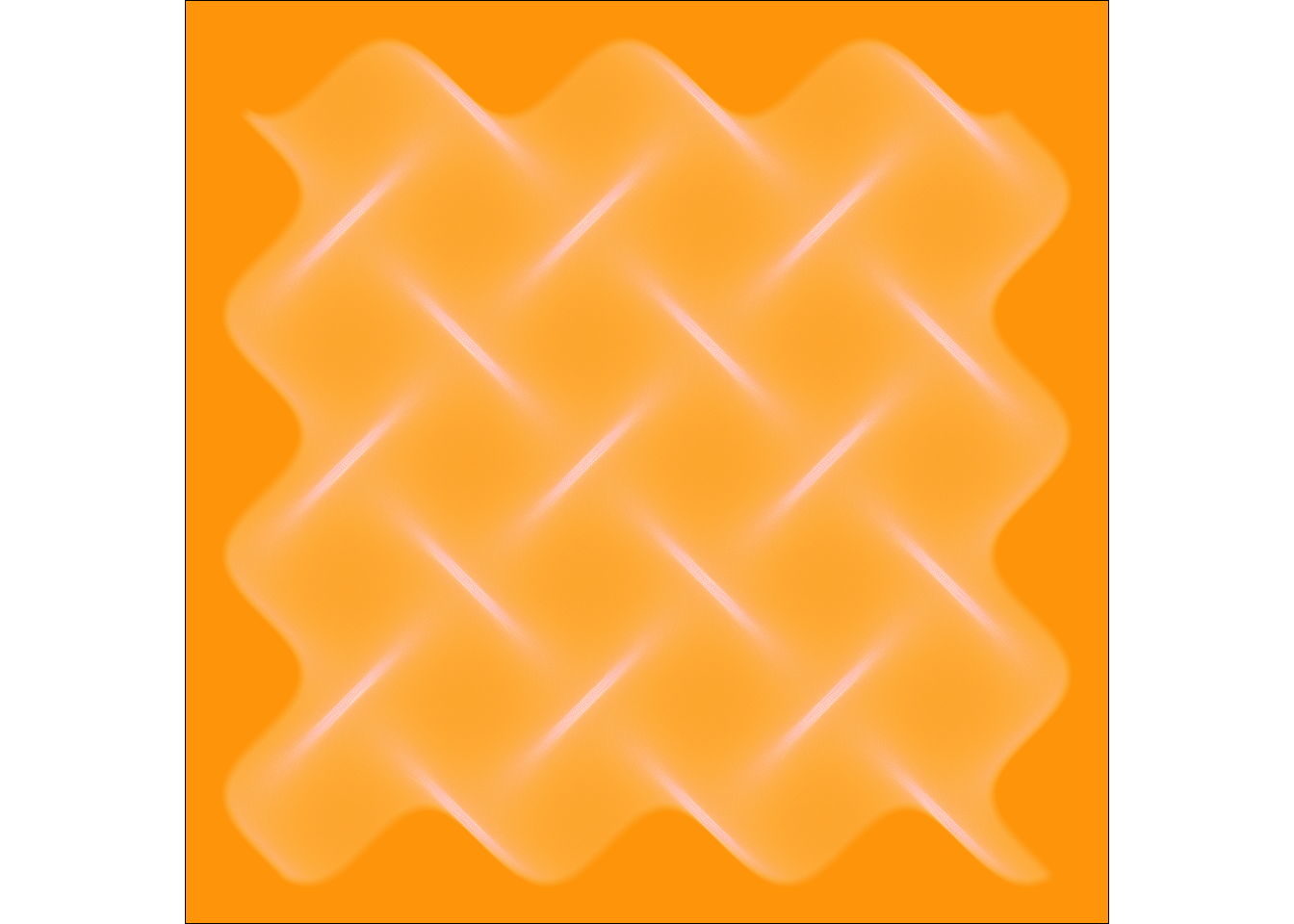``````## Wave Weave

ggplot() +
geom_point(data = kw.seq,
mapping = aes(x = ((x*x) + sin(y)), y = ((y*y) + cos(x))),
alpha = 0.01, colour = "white") +
coord_fixed() +
theme_void() +
theme(panel.background = element_rect(fill = "blue"))````````````  ## Multiple Square Panel Weave
ggplot() +
geom_point(data = kw.seq,
mapping = aes(x = (sin(x) + cos(x)), y = (sin(y) + cos(y))),
alpha = 0.02, colour = "white") +
geom_point(data = kw.seq,
mapping = aes(x = (sin(x) + cos(x) - 2), y = (sin(y) + cos(y) - 2)),
alpha = 0.02, colour = "blue") +
coord_fixed() +
theme_void() +
theme(panel.background = element_rect(fill = "green"))````````````  ## Square tan Weave
ggplot() +
geom_point(data = kw.seq,
mapping = aes(x = (2*x + tan(y)), y = (2*y + tan(x))),
alpha = 0.02, colour = "white") +
ylim(-50, 50) + xlim(-50, 50) +
coord_fixed() +
theme_void() +
theme(panel.background = element_rect(fill = "green"))``````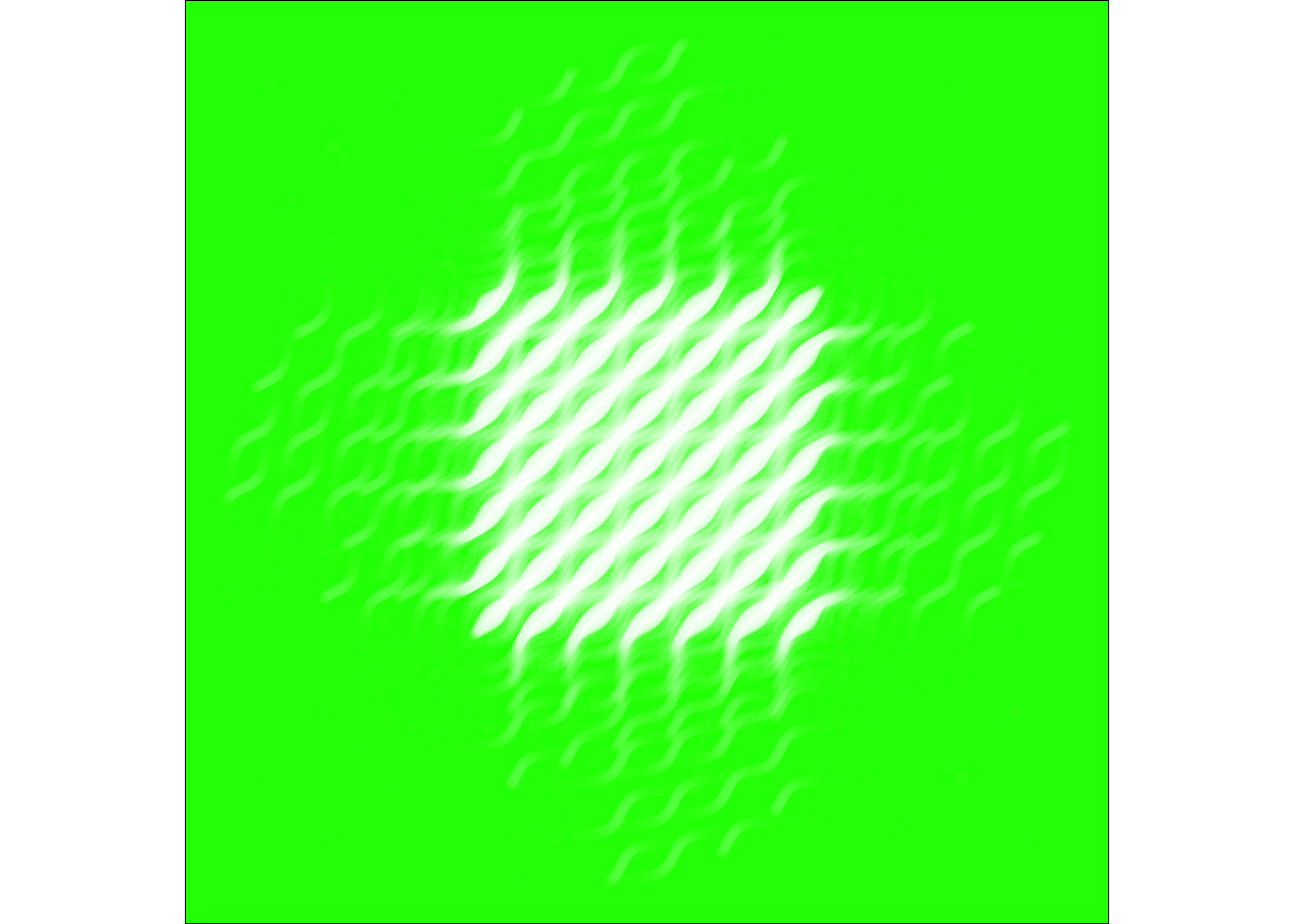``````## Jellyfish

ggplot() +
geom_point(data = kw.seq,
mapping = aes(x = (sqrt(x) + (sin(y))), y = (sqrt(y) + (sin(x)))),
alpha = 0.1, colour = "blue") +
coord_fixed() +
theme_void() +
theme(panel.background = element_rect(fill = "orange"))``````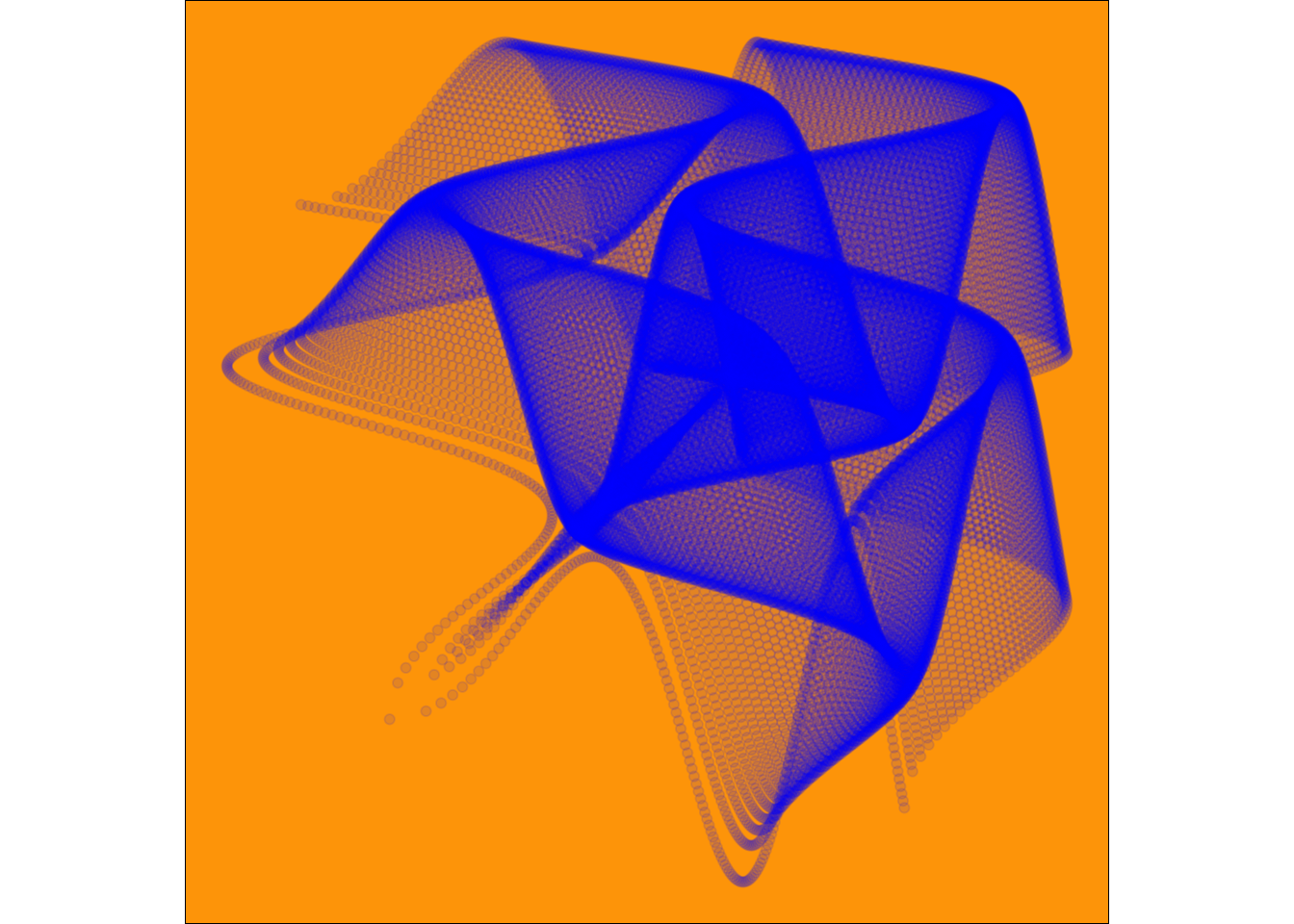``````## Random Square

ggplot() +
geom_point(data = (kw.seq + 25),
mapping = aes(x = (sqrt(x) + (sin(y))), y = (sqrt(y) + (sin(x)))),
alpha = 0.02, colour = "blue") +
coord_fixed() +
theme_void() +
theme(panel.background = element_rect(fill = "lightpink"))``````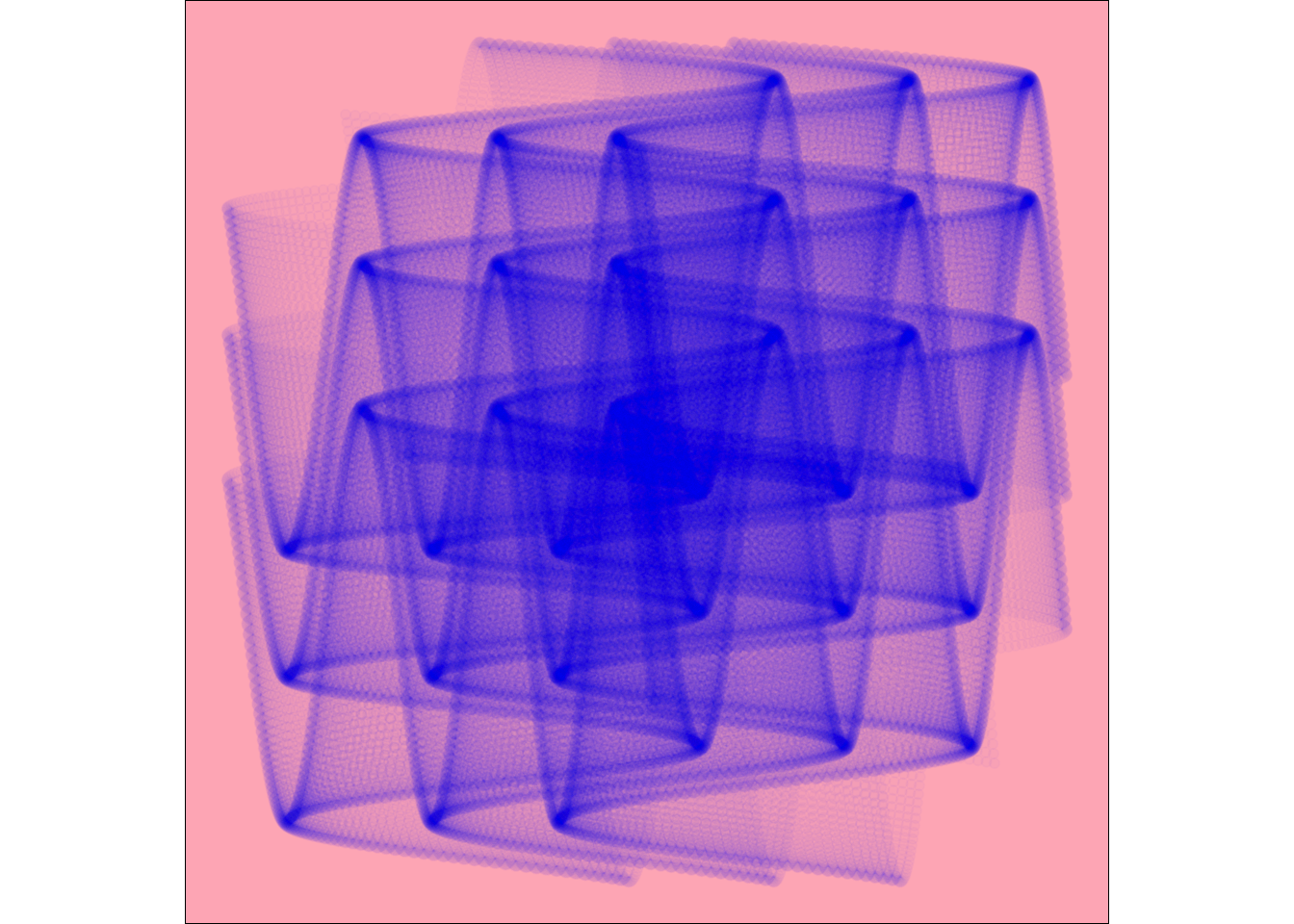``````##
ggplot() +
geom_point(data = (kw.seq),
mapping = aes(x = (cos(x^2)^2 + sin(y)), y = (cos(y^2)^2 + sin(x)),
colour = x, alpha = y)) +
geom_point(data = (kw.seq),
mapping = aes(x = (cos(x^2)^2 + sin(y)), y = (cos(y^2)^2 + sin(x)),
colour = -x, alpha = -y)) +

coord_fixed() +
theme_void() +
theme(panel.background = element_rect(fill = "blue"),
legend.position = "none")``````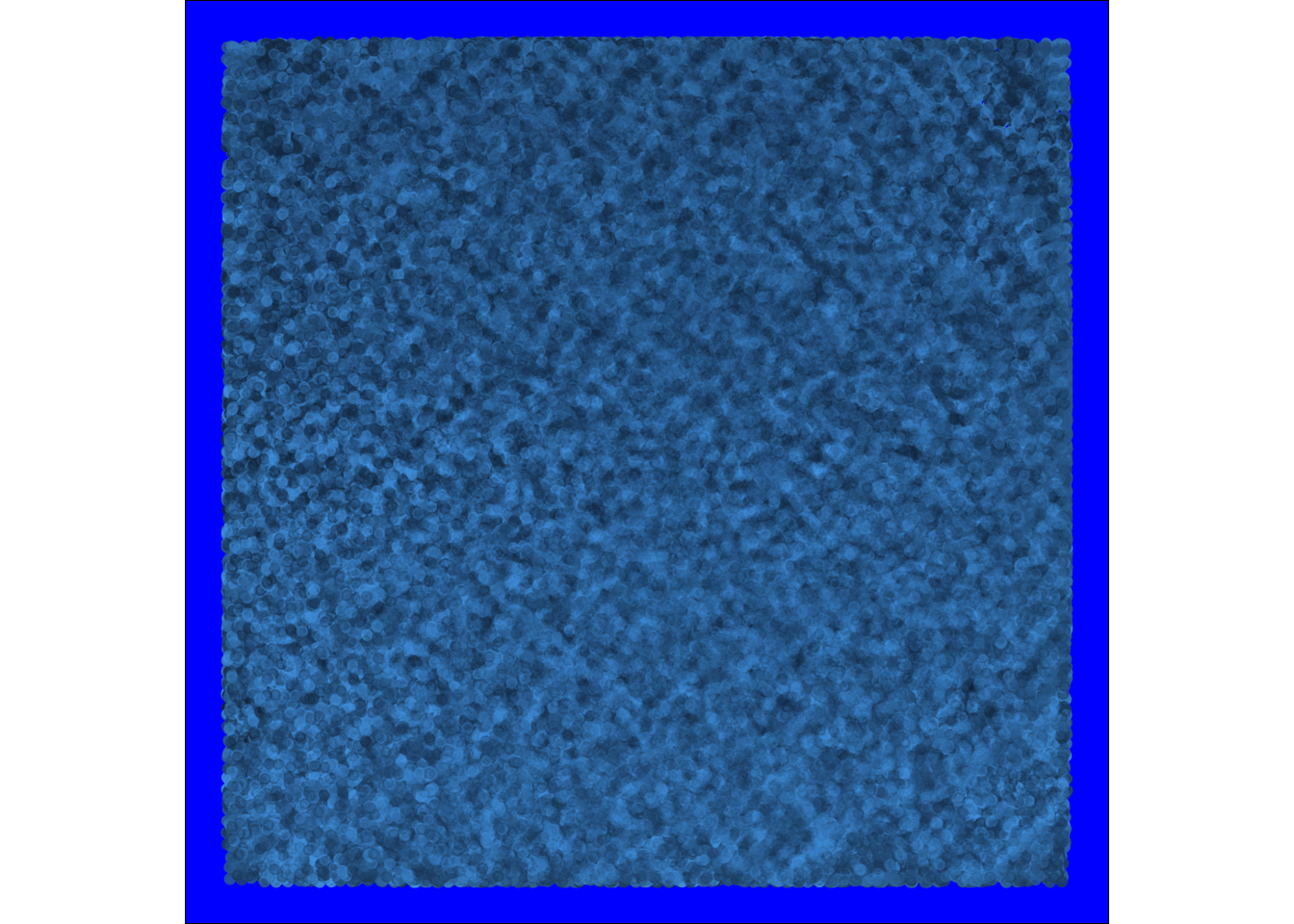Complex Designs

Based on Fronkonstin’s blog

Summoning Cthulhu

``````sc.seq <- seq(from = -3,
to = 3,
by = 0.01) %>%
expand.grid(x = ., y = .)

ggplot(data = sc.seq,
mapping = aes(x=(x^3-sin(y^2)), y=(y^3-cos(x^2)))) +
geom_point(alpha=.1, shape=20, size=0, color="white") +
theme_void() +
theme(panel.background = element_rect(fill="black")) +
coord_polar()``````Marine Creature

``````mc.seq <- seq(from = -10,
to = 10,
by = 0.05) %>%
expand.grid(x = ., y = .)

ggplot(data = mc.seq,
mapping = aes(x=(x^2+pi*cos(y)^2), y=(y+pi*sin(x)))) +
geom_point(alpha=.1, shape=20, size=1, color="black")+
theme_void()+coord_fixed()``````